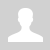Python. Polymorphism (En)
 11.4.2.1 explain the concept of polymorphism with examples Python. Polymorphism Polymorphism - different behavior of the same method in different classes. Polymorphism is defined by a function that can process data of different types. The property of code to work with different data types is called polymorphism. Examples One example is the function len(). We can define the length of string, list, tuple, etc. ```print(len('school'))  # 6 print(len([2, 3, 5, 7, 11, 13, 17, 19, 23]))  # 9 print(len(('A', 'B', 'C')))  # 3``` Other example, operation + (addition). In Python we can add whole numbers, float numbers, strings, lists, etc. ```print(5 + 3)                    # 8 print(2.7 + 3.4)                # 6.1 print('Hello, ' + 'world!')     # Hello, world! print([2, 3, 5] + [7, 11, 13])  # [2, 3, 5, 7, 11, 13]``` Use addition function ```def func_add(x, y):     return x + y print(func_add(5, 3))                    # 8 print(func_add(2.7, 3.4))                # 6.1 print(func_add('Hello, ', 'world!'))     # Hello, world! print(func_add([2, 3, 5], [7, 11, 13]))  # [2, 3, 5, 7, 11, 13]``` All function calls work correctly because Python is a dynamically typed language. The interpreter chooses the correct implementation of the operation +. Consider an example of programming code that contains two classes: Circle() and Square(). Each class has methods for measuring area and perimeter. ```from math import pi class Circle:     def __init__(self, radius): # initialize one attribute radius         self.radius = radius        def area(self):         return pi * self.radius ** 2       def perimeter(self):         return 2 * pi * self.radius   class Square:     def __init__(self, side): # initialize one attribute side         self.side = side       def area(self):         return self.side * self.side       def perimeter(self):         return 4 * self.side      def print_shape_info(shape): # polymorphic function for displaying the area and perimeter of a shape     print(f"Area = {shape.area()}, perimeter = {shape.perimeter()}.") # create an instance square square = Square(2) print_shape_info(square)   # Area = 4, perimeter = 8. # create an instance circle circle = Circle(5) print_shape_info(circle)     # Area = 78.53981633974483, perimeter = 31.41592653589793.```   Questions: Explain the term "polymorphism". Give an example of polymorphism in real life. How to find out by the class name what methods it implements? Exercises: Tasks: .u-star-rating-13 { list-style:none; margin:0px; padding:0px; width:65px; height:13px; position:relative; background: url('/.s/t/2001/rating13.png') top left repeat-x } .u-star-rating-13 li{ padding:0px; margin:0px; float:left } .u-star-rating-13 li a { display:block;width:13px;height: 13px;line-height:13px;text-decoration:none;text-indent:-9000px;z-index:20;position:absolute;padding: 0px;overflow:hidden } .u-star-rating-13 li a:hover { background: url('/.s/t/2001/rating13.png') left center;z-index:2;left:0px;border:none } .u-star-rating-13 a.u-one-star { left:0px } .u-star-rating-13 a.u-one-star:hover { width:13px } .u-star-rating-13 a.u-two-stars { left:13px } .u-star-rating-13 a.u-two-stars:hover { width:26px } .u-star-rating-13 a.u-three-stars { left:26px } .u-star-rating-13 a.u-three-stars:hover { width:39px } .u-star-rating-13 a.u-four-stars { left:39px } .u-star-rating-13 a.u-four-stars:hover { width:52px } .u-star-rating-13 a.u-five-stars { left:52px } .u-star-rating-13 a.u-five-stars:hover { width:65px } .u-star-rating-13 li.u-current-rating { top:0 !important; left:0 !important;margin:0 !important;padding:0 !important;outline:none;background: url('/.s/t/2001/rating13.png') left bottom;position: absolute;height:13px !important;line-height:13px !important;display:block;text-indent:-9000px;z-index:1 } Категория: Programming languages | Добавил: bzfar77 (11.04.2022) Просмотров: 538 | Теги: polymorphism, Class, Attribute, Python, Method | Рейтинг: 5.0/1
 Всего комментариев: 0